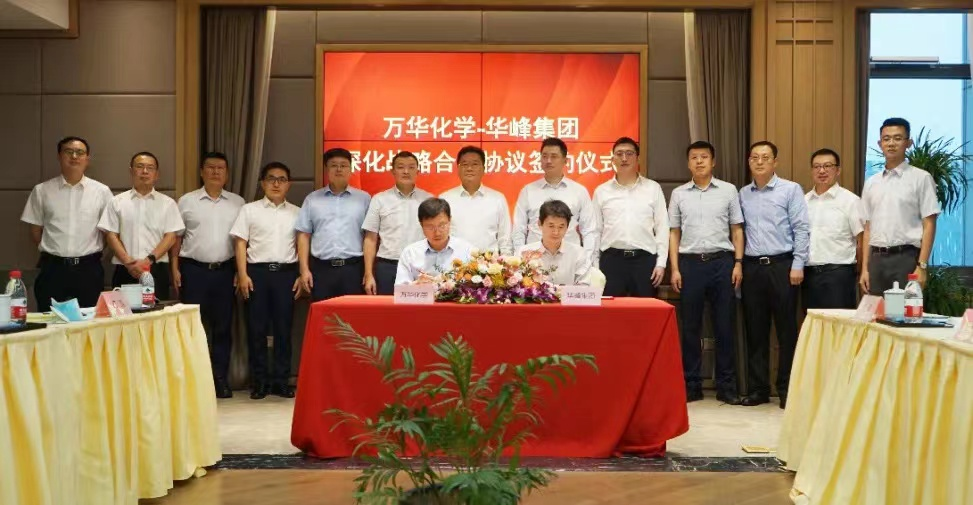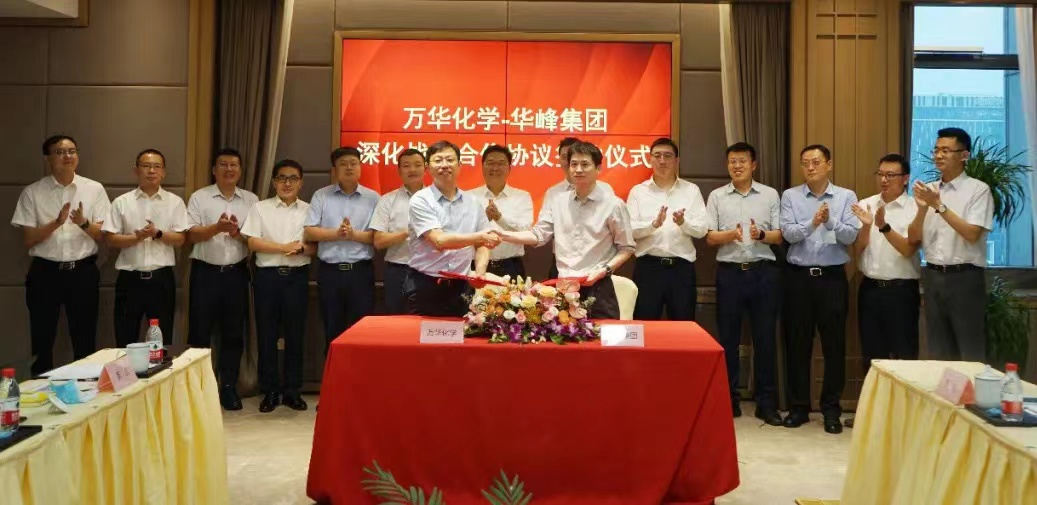{msg=success, data={columnId=100, navBreadcrumb=[{brother=[], entityTag=, id=null, name=新闻中心, type=false, url=/column/100/}, {brother=[], entityTag=, id=null, name=深化合作 携手共赢 | 万华化学与华峰集团续签战略合作协议, type=false, url=}], list=[{columnId=76, name=首页, linkUrl=}, {columnId=1, name=关于万华, linkUrl=/column/79/}, {columnId=3, name=产品与行业解决方案, linkUrl=/column/80/}, {columnId=2, name=可持续发展, linkUrl=/column/12/}, {columnId=100, name=新闻中心, linkUrl=/column/100/}, {columnId=84, name=投资者关系, linkUrl=/column/84/}, {columnId=6, name=产品热线, linkUrl=}, {columnId=87, name=站点/语言维护, linkUrl=}, {columnId=152, name=法律声明, linkUrl=/cmscontent/432.html}, {columnId=153, name=隐私保护, linkUrl=/cmscontent/433.html}, {columnId=262, name=廉洁从业, linkUrl=}], homePage={brother=[], entityTag=, id=null, name=Home_2, type=false, url=/}}, status=101}

# 深化合作 携手共赢 | 万华化学与华峰集团续签战略合作协议